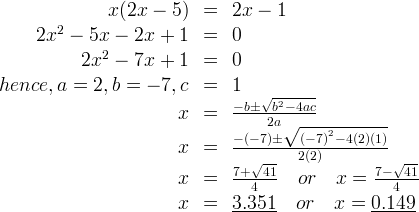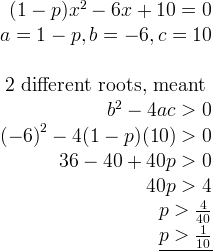# SPM Questions for Quadratic Equations

We will discuss some pass year SPM exam questions based on:

• Solve the quadratic equations – SPM 2003, SPM 2005
• Form a quadratic equation – SPM 2009
• Determine the conditions for the type of roots – SPM2010

### Solve the quadratic equations – SPM 2003 Paper 1 Question 3

(3 marks)Note: If you have any doubt, please refer back my previous post on how to solve the equation using completing the square method.

### SPM 2005 Paper1 Question 5

(3 marks)### Form a quadratic equation – SPM 2009 Paper 2 Question 2

The quadratic equation x2 – 5x + 6 = 0 has roots h and k, where h > k.

(a) Find

i) the value of h and of k.
ii) the range of x if x2 – 5x + 6 > 0
(5 marks)

(b) Using the values of h and k from 2(a)(i), form the quadratic equation which has roots h + 2 and 3k – 2.
(2 marks)

a i) For x– 5x + 6 = 0, given roots are h and k,ii)(b)$h + 2$$= 3 + 2$$= 5$$3k - 2$$= 3(2) - 2$$= 4$$SOR = 5 + 4 = 9$$\text{POR = 5 x 4 = 20}$$Equations:$${{x}^{2}}-(\text{SOR)}x+(\text{POR)}=0$$\underline{{{x}^{2}}-9x+20=0}$

### Determine the conditions for the type of roots – SPM 2010 Paper1 Question 5

The quadratic equation (1-p)x2 – 6x +10 = 0, where p is a constant, has two different roots. Find the range of values of p.

(3 marks)